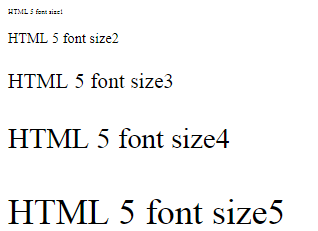### Arithmetic Operators in JavaScript

JavaScript simplifies the use of arithmetic operators as shown in this table:

```
Operator		Sample expression						Meaning
-=				x-= 4							x = x - 4
+=				x+= 4							x = x + 4
*=				x*= 4							x = x * 4
/=				x/= 4							x = x / 4
%=				x%= 4							x = x % 4
--				x--						postdecrement: use the value of x and then decrement by 1
--				--x						predecrement: decrement by 1 and then use the value of x
++				x++						postincrement: use the value of x and then increment by 1
++				++x						preincrement: increment by 1 and the use the value of x
```

### Loop: Example

Another example of using loops in JavaScript is shown in the following program:
```	<!DOCTTYPE html>
<!--Loop3.html -->
<html>
<head>
<title> Loop for 5 times </title>
<script >
<!--
var counter; //counter
for (counter = 1; counter <=5; ++counter) //begining of the foor loop
{
document.writeln("< p style = 'font-size: " + counter + "ex'>HTML 5 font size" +
counter + "< p>");
} //end of foor
// -->
</script>
</head>
<body></body>
</html>
```
This figure shows the output of the above JavaScript program and how the Forloop iterates a number of times:For more details, please contact me here.
Date of last modification: March 26, 2019.